0%

p.s. 啃书太累了，尤其是对于自己来说相对还算陌生领域的内容。本人在游戏行业待了10年，转研发也有一半的时间了，对于基础的3D知识也算了解，但啃完一章也得半个多月。原书中一章可能就短短50页的内容，但其中某句话中涉及的一个专业词汇就得花至少半天时间去查资料看视频才能了解，再加上我不想随便机翻一下英文就当读书笔记，想要把内容吃透后用自己的语言写下来，就更是难上加难了。难怪不是水货的TA壁垒这么高。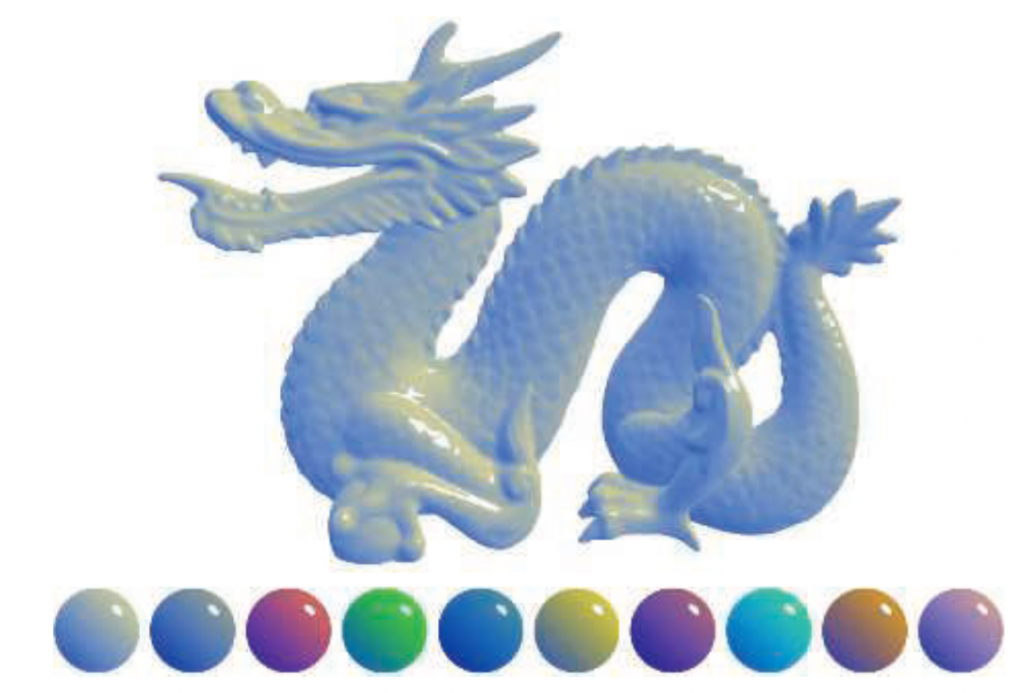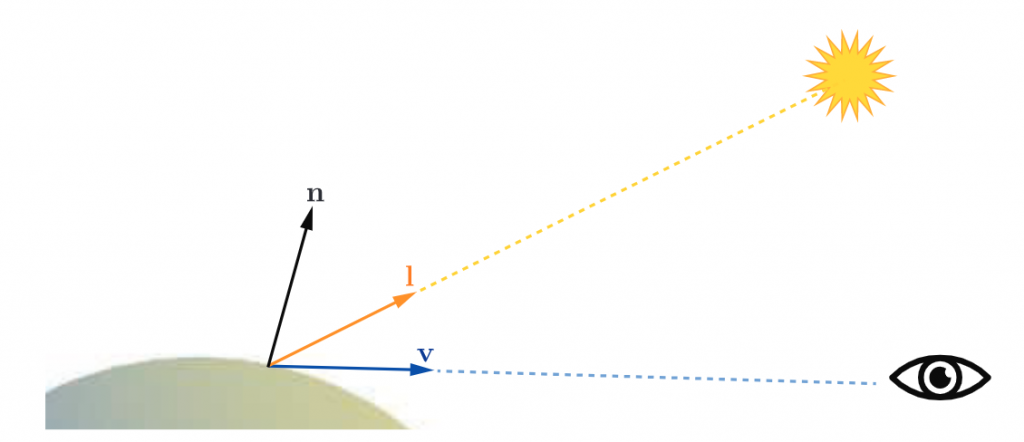$\mathbf{c}_{shaded}=s\mathbf{c}_{highlight}+(1-s)(t\mathbf{c}_{warm}+(1-t)\mathbf{c}_{cool})$

$\mathbf{c}_{\text{cool}}=(0,0,0.55)+0.25\mathbf{c}_{\text{surface}},$

$\mathbf{c}_{\text{warm}}=(0.3,0.3,0)+0.25\mathbf{c}_{\text{surface}},$

$\mathbf{c}_{\text{highlight}}=(1,1,1),$

$t=\frac{(\mathbf{n} \cdot \mathbf{l})+1}{2},$

$\mathbf{r}=2(\mathbf{n} \cdot \mathbf{l})\mathbf{n}-\mathbf{l},$

$s=(100(\mathbf{r} \cdot \mathbf{v})-97)^{\mp}$

$\mathbf{r}=2(\mathbf{n} \cdot \mathbf{l})\mathbf{n}-\mathbf{l}$用来计算l相对n的反射光。这在shader中也是一个内置函数，reflect。

### 光源(Light Sources)

$\mathbf{c}_{\text{shaded}}=f_{\text{unlit}}(\mathbf{n},\mathbf{v})+k_{\text{light}}f_{\text{lit}}(\mathbf{l},\mathbf{n},\mathbf{v})$

$\mathbf{c}_{\text{shaded}}=f_{\text{unlit}}(\mathbf{n},\mathbf{v})+\mathbf{c}_{\text{light}}f_{\text{lit}}(\mathbf{l},\mathbf{n},\mathbf{v})$

$\mathbf{c}_{\text{shaded}}=f_{\text{unlit}}(\mathbf{n},\mathbf{v})+\sum_{n=1}^{i}\mathbf{c}_{\text{light}_{i}}f_{\text{lit}}(\mathbf{l}_i,\mathbf{n},\mathbf{v})$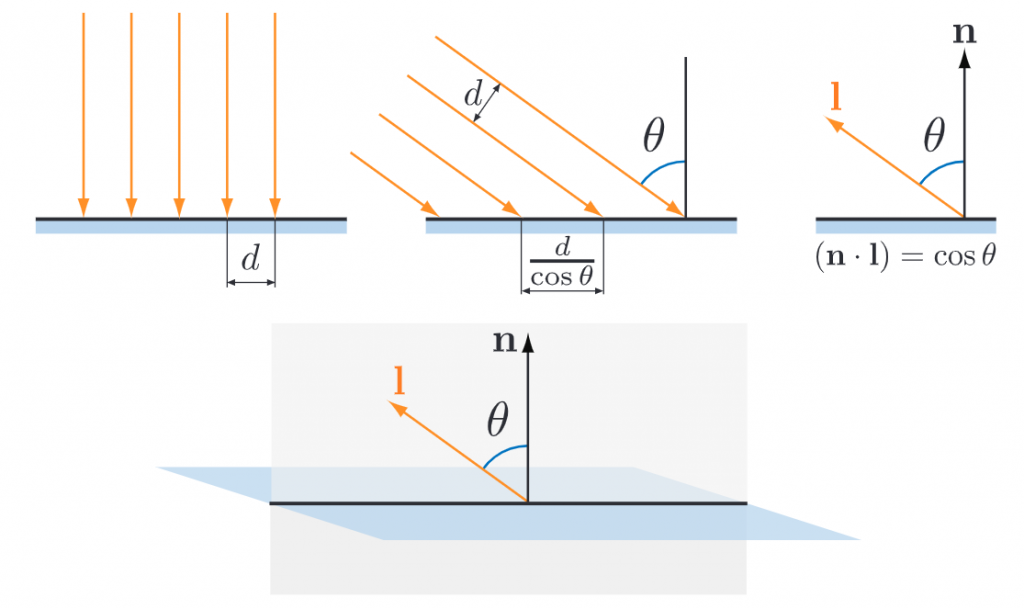$\mathbf{c}_{\text{shaded}}=f_{\text{unlit}}(\mathbf{n},\mathbf{v})+\sum_{n=1}^{i}(\mathbf{l}_i \cdot \mathbf{n})^{+}\mathbf{c}_{\text{light}_{i}}f_{\text{lit}}(\mathbf{l}_i,\mathbf{n},\mathbf{v})$

$f_{\text{lit}}()$最简单的实现方式是使用固定颜色：

$f_{\text{lit}}()=\mathbf{c}_{\text{surface}}$

$\mathbf{c}_{\text{shaded}}=f_{\text{unlit}}(\mathbf{n},\mathbf{v})+\sum_{n=1}^{i}(\mathbf{l}_i \cdot \mathbf{n})^{+}\mathbf{c}_{\text{light}_{i}}\mathbf{c}_{\text{surface}}$

#### 精确光源(Punctual Lights)

$\mathbf{l}=\frac{\mathbf{p}_{\text{light}}-\mathbf{p}_0}{\|\mathbf{p}_{\text{light}}-\mathbf{p}_0 \|}$

$\mathbf{d}=\mathbf{p}_{\text{light}}-\mathbf{p}_0$

$r=\sqrt{\mathbf{d} \cdot \mathbf{d}}$

$\mathbf{l}=\frac{\mathbf{d}}{r}$

##### 点光源(Point/Omni Lights)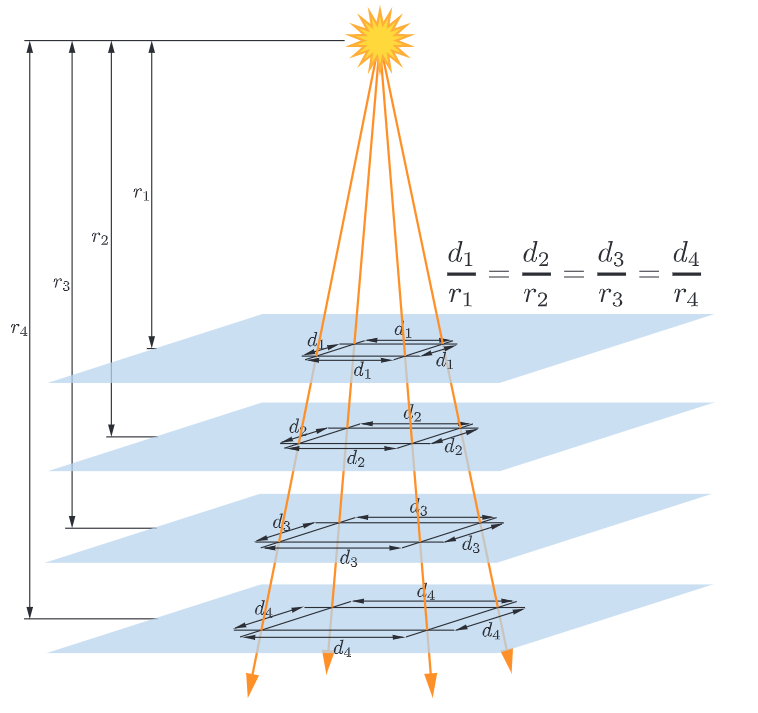$\mathbf{c}_{\text{light}}(r)=\mathbf{c}_{\text{light}_0}(\frac{r_0}{r})^2$

$\mathbf{c}_{\text{light}}(r)=\mathbf{c}_{\text{light}_0}\frac{r_0^2}{r^2+\epsilon}$

$\mathbf{c}_{\text{light}}(r)=\mathbf{c}_{\text{light}_0}(\frac{r_0}{\max (r, r_{\min})})^2$

$f_{\text{win}}(r)=(1-(\frac{r}{r_{\max}})^4)^{+2}$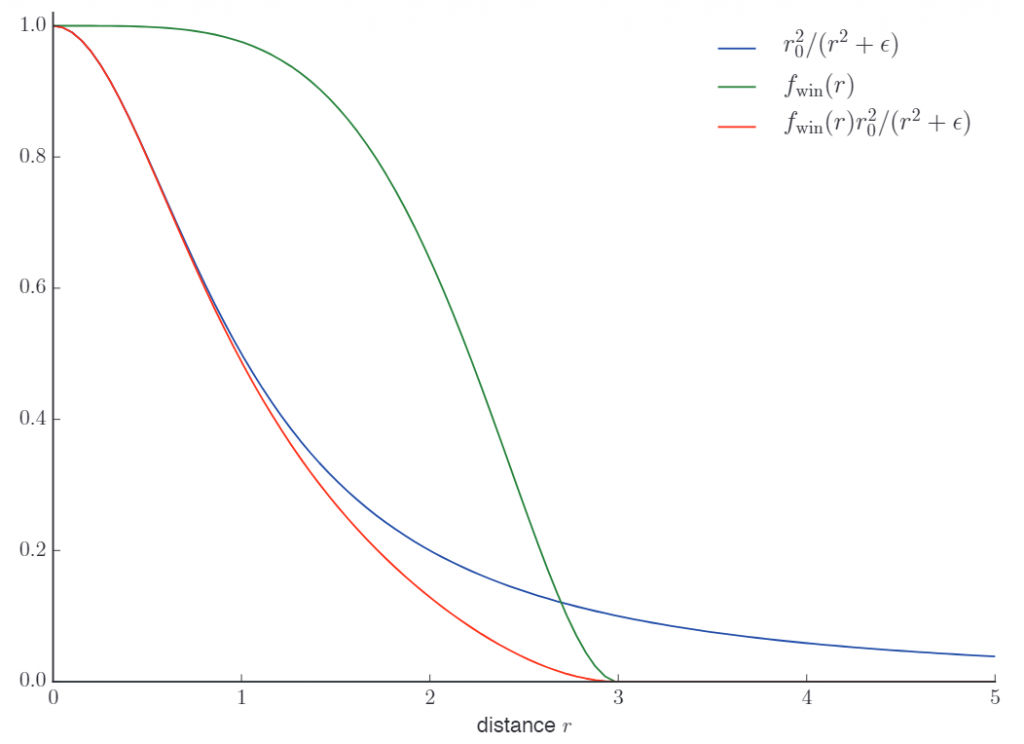$\mathbf{c}_{\text{light}}(r)=\mathbf{c}_{\text{light}_0}f_{\text{dist}}(r)$

$f_{\text{dist}}(r)=(1-(\frac{r}{r_{\max}})^2)^{+2}$

##### 聚光灯(Spotlights)

$\mathbf{c}_{\text{light}}=\mathbf{c}_{\text{light}_0}f_{\text{dist}}(r)f_{\text{dir}}(\mathbf{l})$

$t=(\frac{\cos \theta_s-\cos \theta_u}{\cos \theta_p-\cos \theta_u})^{\mp}\;\;\;\;\;\text{(clamp到0,1之间)}$

$f_{\text{dir}_{\text{F}}}(\mathbf{l})=t^2$

$f_{\text{dir}_{\text{T}}}(\mathbf{l})=\text{smoothstep}(t)=t^2(3-2t)$

#### 其他光源类型

• 模拟区域光被部分遮挡产生的柔和阴影边缘
• 模拟区域光对表面着色的影响

#### 求值频率(Frequency of Evaluation)

• 计算结果会在整个应用运行阶段持续变化，但是变化很慢，完全不需要每一帧都进行更新，比如随着游戏内一天的时间变化而改变的光照因素。如果计算的消耗很高，那可以尝试每隔一些帧数再更新。
• 每一帧都需要进行计算，比如级联视图和透视矩阵
• 针对每个模型需要进行计算，比如更新模型基于位置的光照参数
• 每次draw call进行计算，比如更新模型的材质参数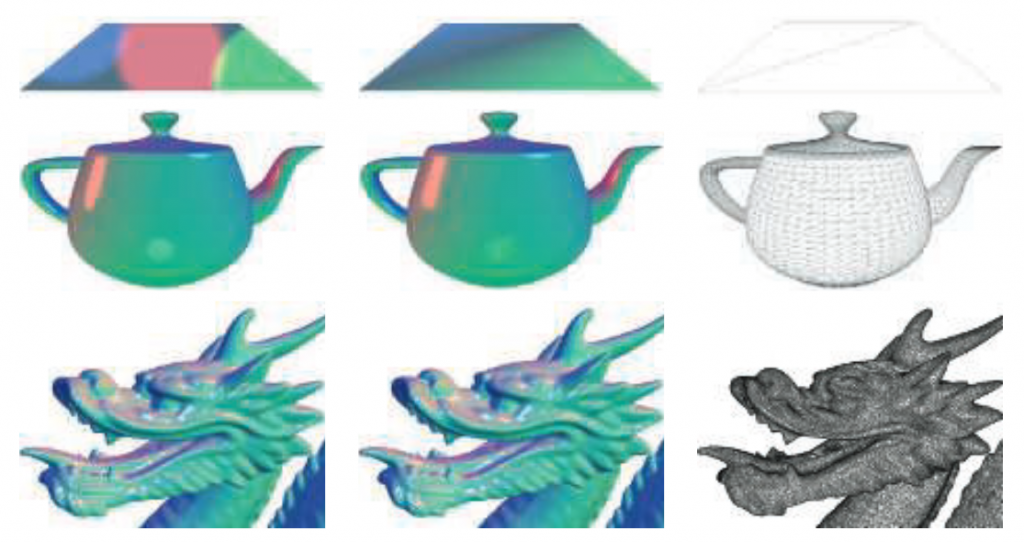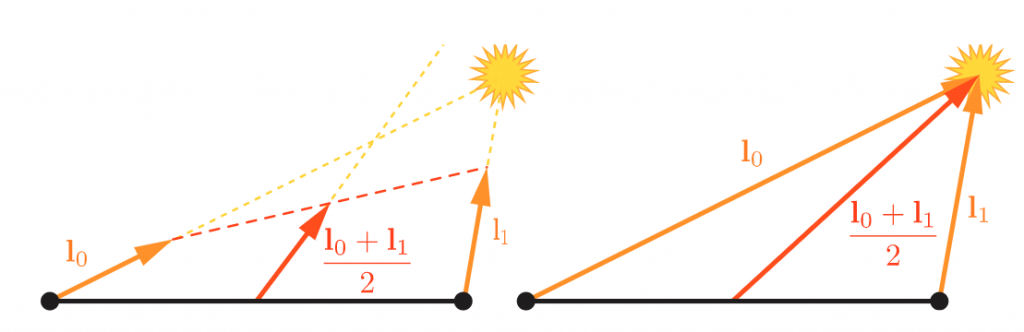#### 例子

$\mathbf{c}_{\text{shaded}}=\frac{1}{2}\mathbf{c}_{\text{cool}}+\sum_{n=1}^{i}(\mathbf{l}_i \cdot \mathbf{n})^{+}\mathbf{c}_{\text{light}_{i}}(s_i\mathbf{c}_{\text{highlight}}+(1-s_i)\mathbf{c}_{\text{warm}})$

$\mathbf{c}_{\text{cool}}=(0,0,0.55)+0.25\mathbf{c}_{\text{surface}},$

$\mathbf{c}_{\text{warm}}=(0.3,0.3,0)+0.25\mathbf{c}_{\text{surface}},$

$\mathbf{c}_{\text{highlight}}=(2,2,2),$

$\mathbf{r}=2(\mathbf{n} \cdot \mathbf{l}_i)\mathbf{n}-\mathbf{l}_i,$

$s=(100(\mathbf{r}_i \cdot \mathbf{v})-97)^{\mp}$

$\mathbf{c}_{\text{shaded}}=f_{\text{unlit}}(\mathbf{n},\mathbf{v})+\sum_{n=1}^{i}(\mathbf{l}_i \cdot \mathbf{n})^{+}\mathbf{c}_{\text{light}_{i}}f_{\text{lit}}(\mathbf{l}_i,\mathbf{n},\mathbf{v})$

$f_{\text{unlit}}(\mathbf{n},\mathbf{v})=\frac{1}{2}\mathbf{c}_{\text{cool}}$

$f_{\text{lit}}(\mathbf{l}_i,\mathbf{n},\mathbf{v})=s_i\mathbf{c}_{\text{highlight}}+(1-s_i)\mathbf{c}_{\text{warm}}$

#### 材质系统(Material Systems)

• 代码复用：将常用方法放到共享文件中，通过#include调用
• 基于模板：定义一个接口，只要某个功能实现符合这个接口规范，就能将其加入进来。这种接口的一个常见用法是将shader的参数计算和shader本身的计算分离开。UE中就有不同的material domain，比如surface domain用来计算shader的参数，Light Function domain用来计算某个光源的$\mathbf{c}_{\text{light}}$值。Unity中也有类似的结构，叫做surface shader。延迟渲染强制使用类似的结构，它使用G-buffer作为接口。

### 混叠和抗锯齿(Aliasing and Antialiasing)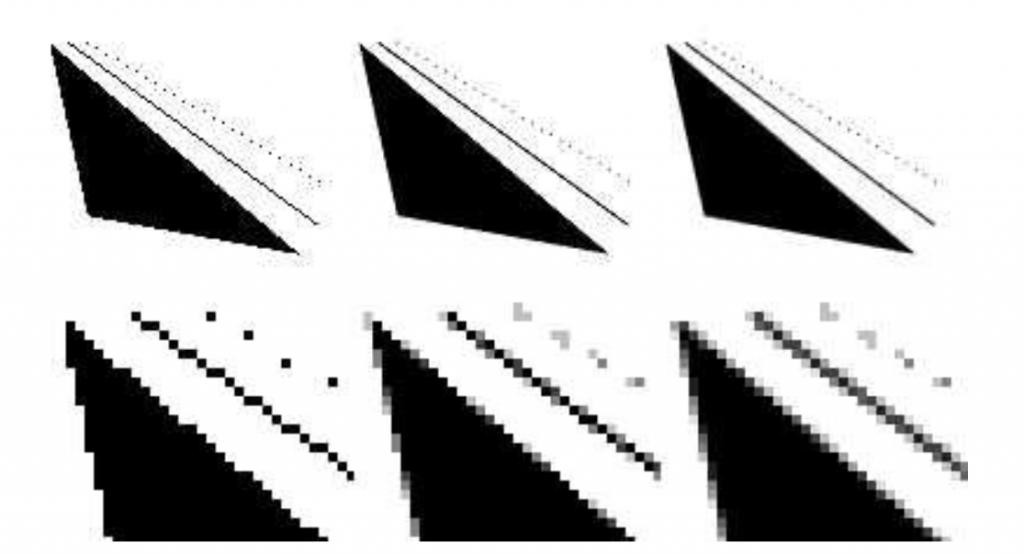#### 采样和过滤理论(Sampling and Filtering Theory)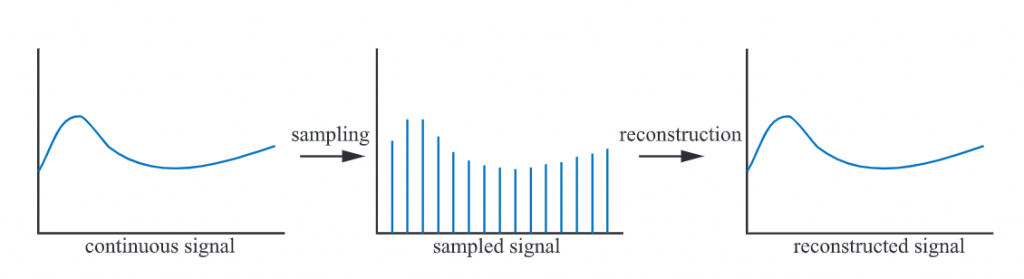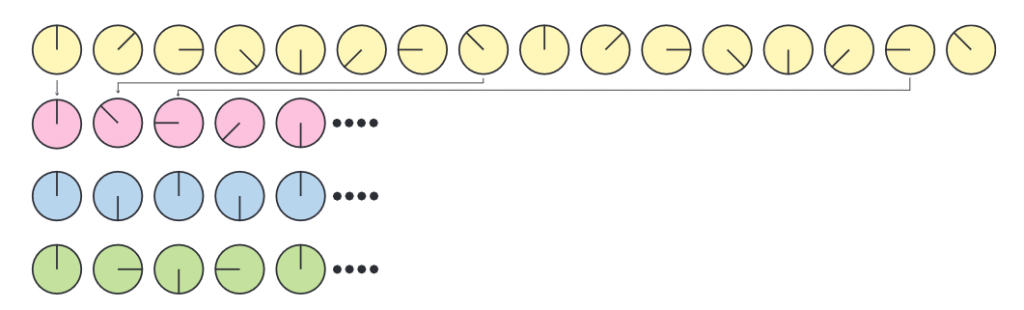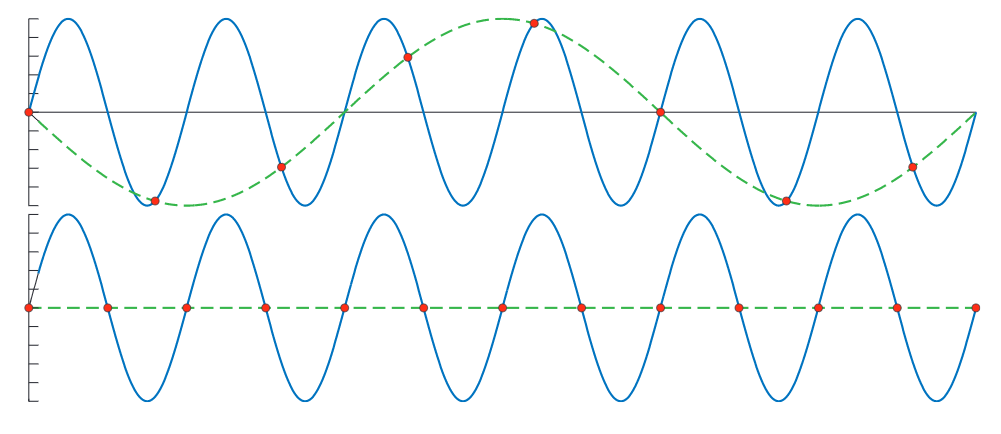##### 重构(reconstruction)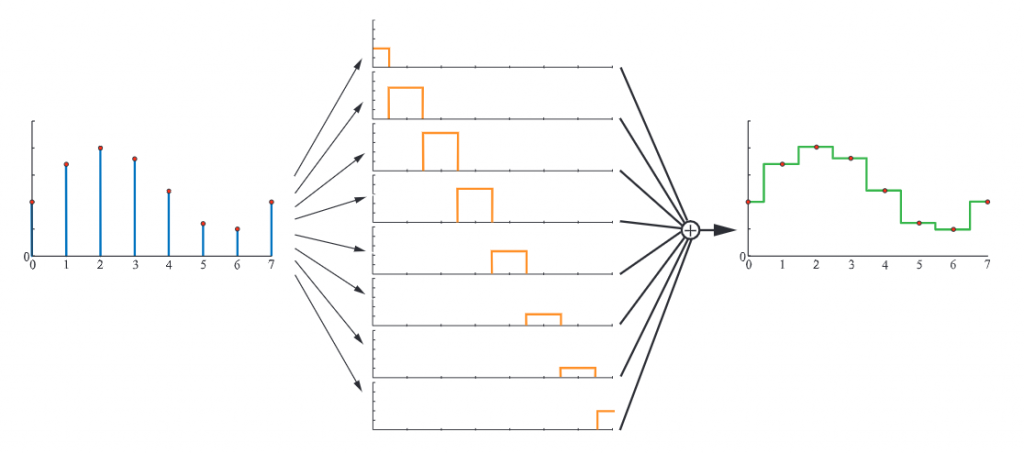box filter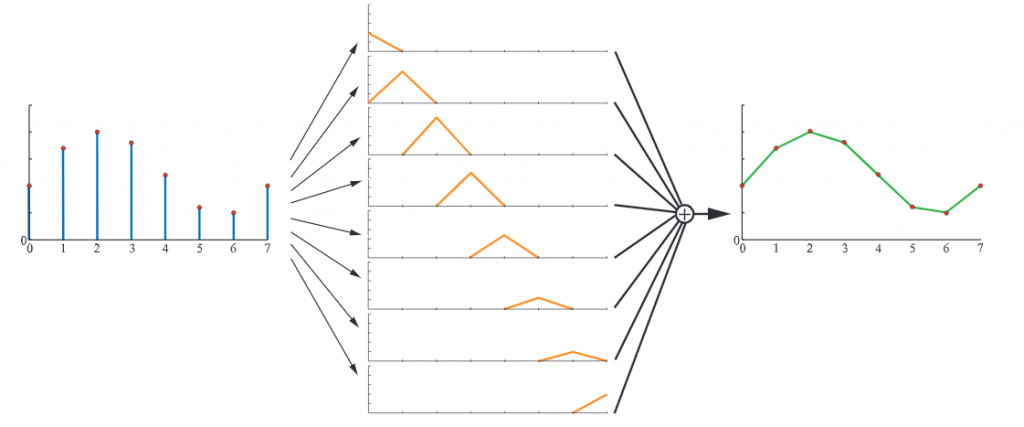tent filter

$\text{sinc}(x)=\frac{\sin(\pi x)}{\pi x}$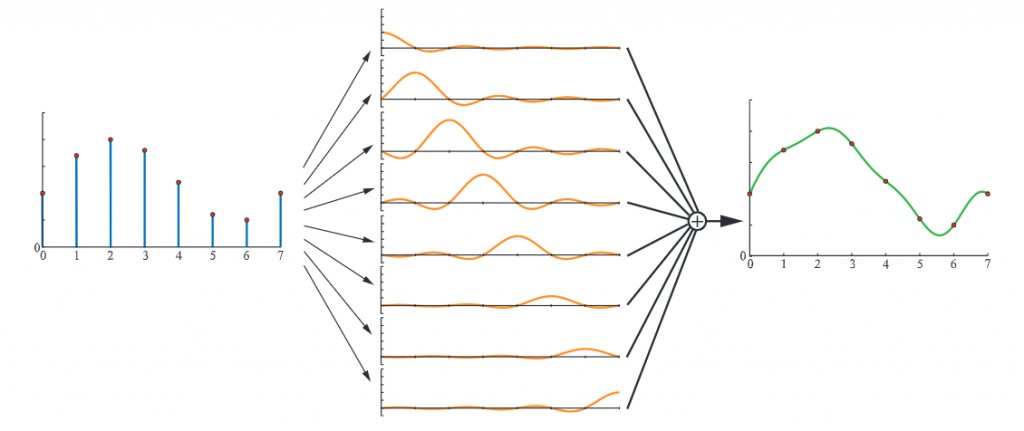sinc filter在采样频率为1时(即原始信号最大频率不高于1/2时)最完美。如果采样频率为$f_s$，则理想的公式为$\text{sinc}(f_sx)$，它将滤去所有频率高于$f_s/2$的部分。

##### 重采样(resampling)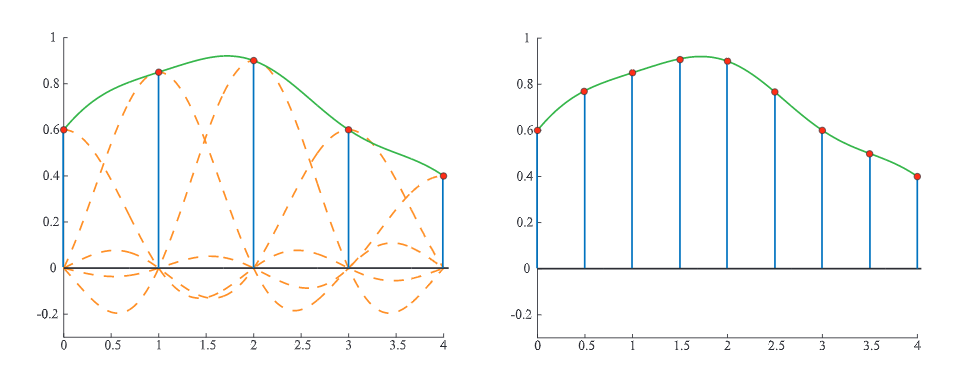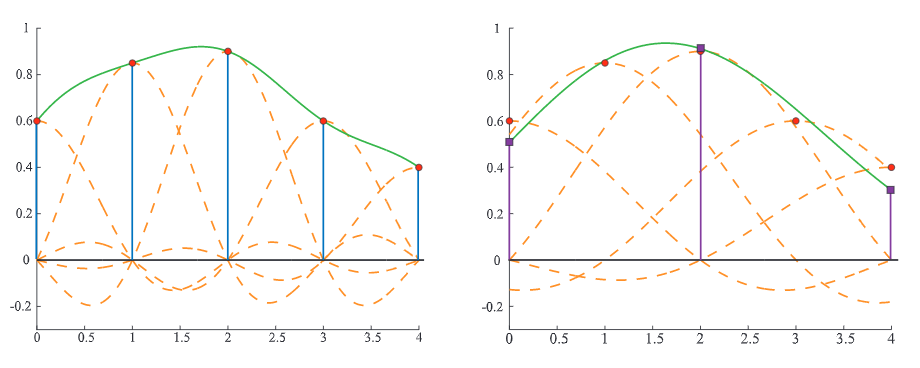### 屏幕空间抗锯齿(Screen-based Antialiasing)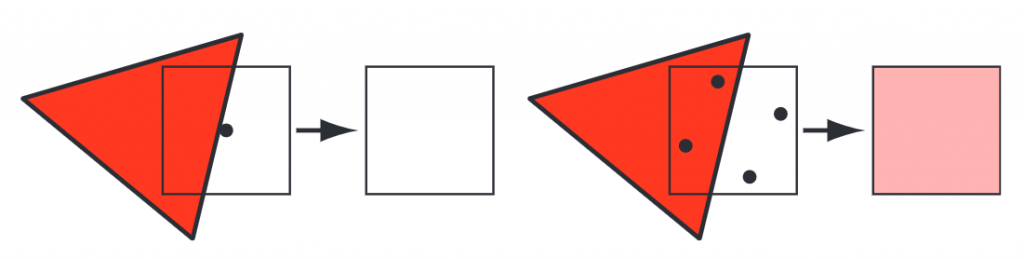$\mathbf{p}(x,y)=\sum_{i=1}^{n}w_i\mathbf{c}(i,x,y)$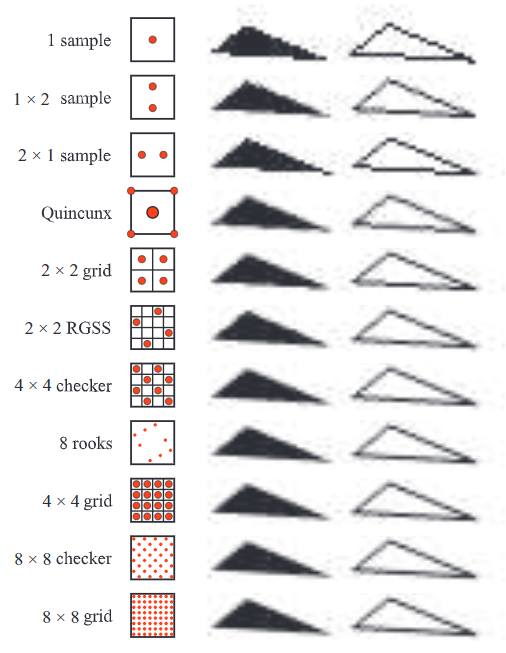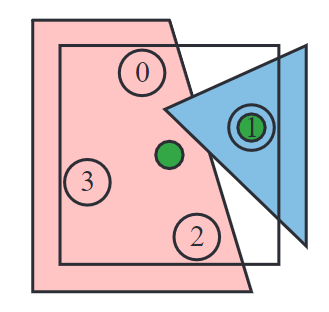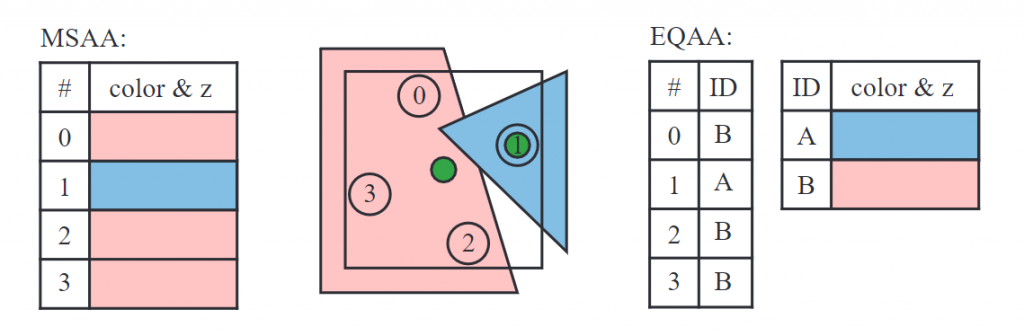##### 采样模式(Sampling Patterns)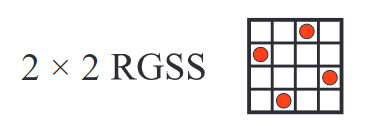RGSS是拉丁超立方(Latin hypercube)采样或N-rook采样的一种形式，也即在$n \\times n$的格子中放n个采样点，每一行每一列都仅有一个采样点。这种采样模式很容易识别接近水平或竖直的边缘。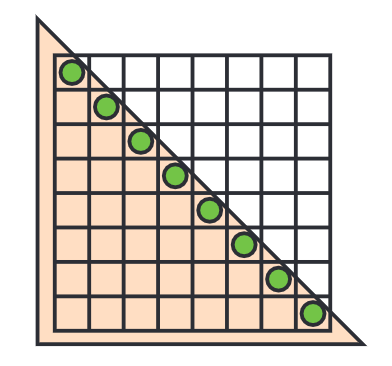GPU生产商在制造硬件时就将采样模式固定了下来。下图是实际中常用的一些采样模式：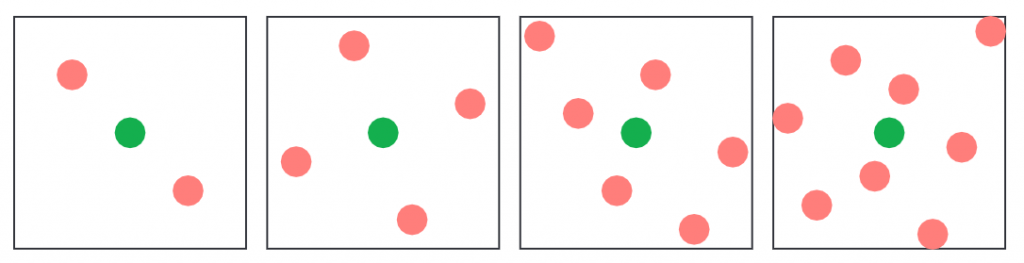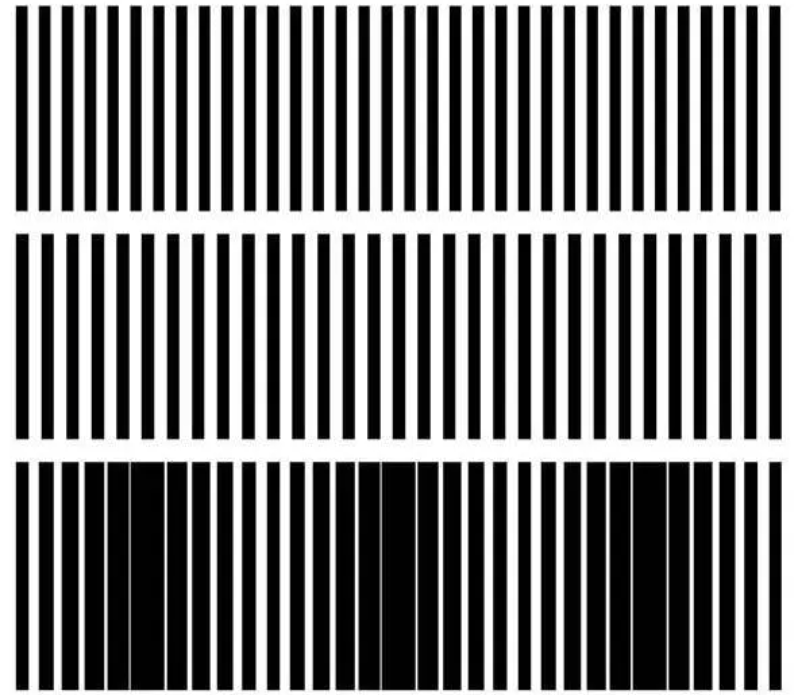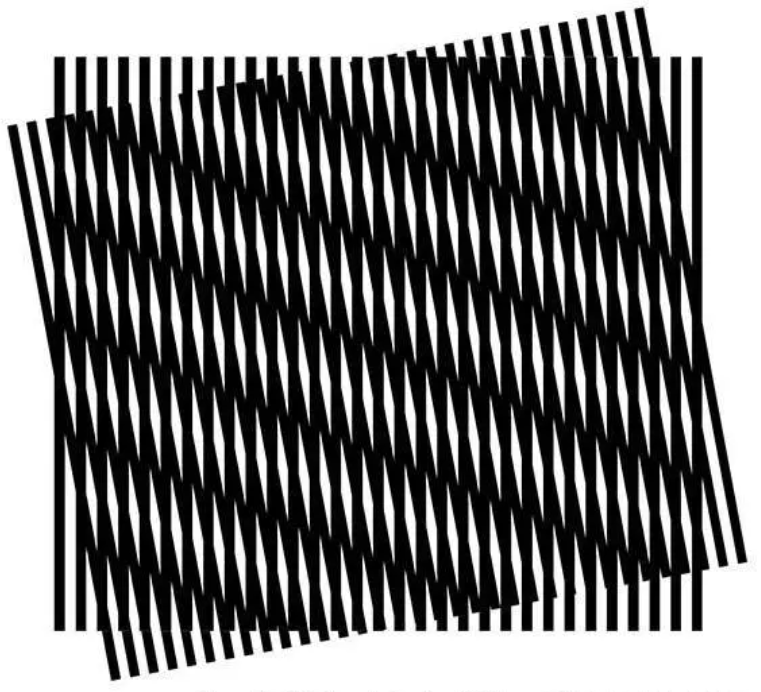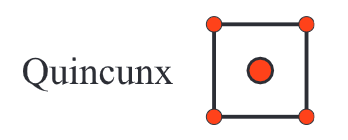Quincunx同样能应用于TAA。这种情况下，每个像素只需要一个采样点，每一帧都会在上一帧基础上往某个方向交替偏移半个像素，这样前一帧的相邻2x2像素在经过双线性插值后(bilinear interpolation)，就能作为当前帧某个像素四个角上的采样点结果，这个结果会和当前帧的结果再做一次平均。如下图所示：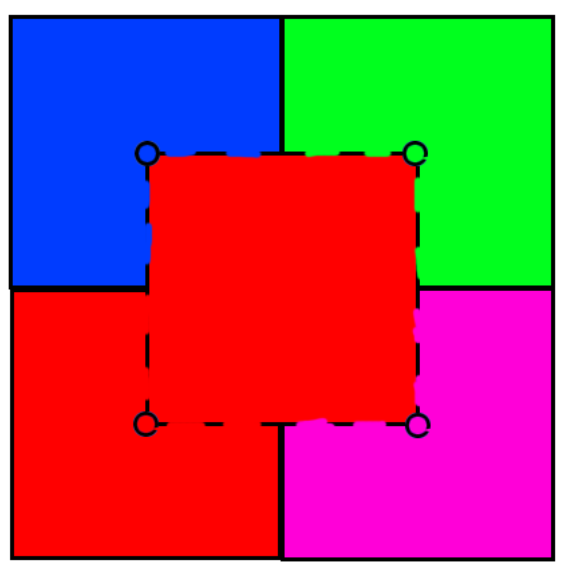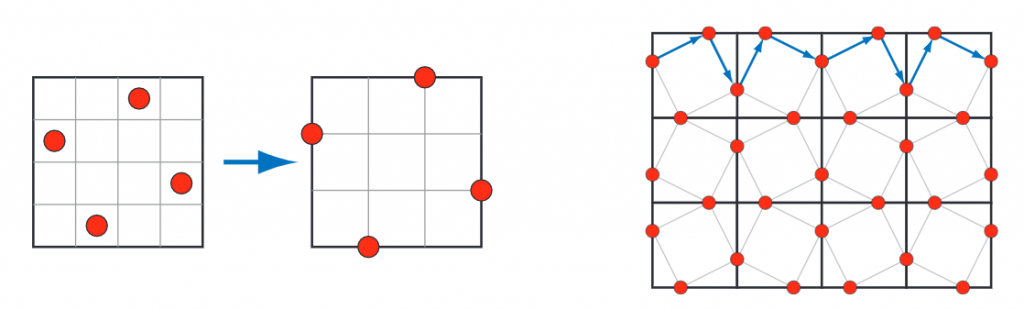##### 形态学方法(Morphological Methods)

MLAA属于后处理，即先按传统方式进行渲染，再额外进行一步抗锯齿操作。有一些方法比如SRAA(subpixel reconstruction antialiasing)依赖于深度值和法线buffer，可以得到更好的效果，但是只适用于几何体。还有一些方法如GBAA(geometry buffer antialiasing)和DEAA(distance-to-edge antialiasing)会计算边缘距离像素中心有多远。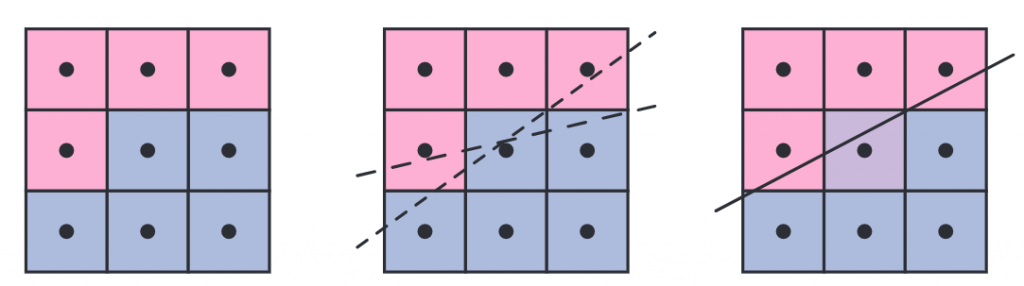### 透明、Alpha和混合(Transparency, Alpha and Compositing)

#### 混合顺序(Blend Order)

$\mathbf{c}_o=\alpha_s\mathbf{c}_s+(1-\alpha_s)\mathbf{c}_d$

over操作在模拟薄纱时效果不错，但是在模拟有色玻璃或塑料时效果就不是很好。被有色玻璃或塑料遮挡的物体应该看起来更暗，因为物体反射的光线只有很少能穿过它们，如下图所示：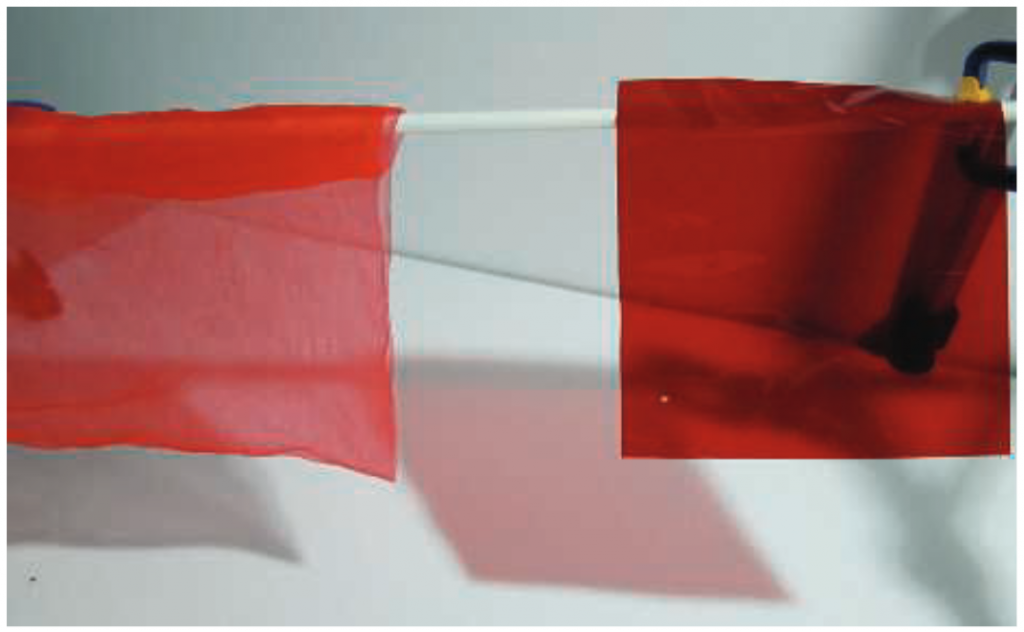$\mathbf{c}_o=\alpha_s\mathbf{c}_s+\mathbf{c}_d$

z-buffer的缺点是一个像素只能存储一个物体。如果一个像素被多个半透明物体覆盖，就会出现问题。在使用over操作时，必须按照从后往前的顺序渲染物体。其中一种排序方式是比较物体质心和观察点的距离。但是这种粗略的排序在有些情况下也会出现问题，如果物体相互穿插，就可能出现远处物体排在近处物体前面的情况。

$\mathbf{c}_o=\alpha_d\mathbf{c}_d+(1-\alpha_d)\alpha_s\mathbf{c}_d$

$\mathbf{a}_o=\alpha_s(1-\alpha_d)+\alpha_d=\alpha_s+\alpha_d-\alpha_s\alpha_d$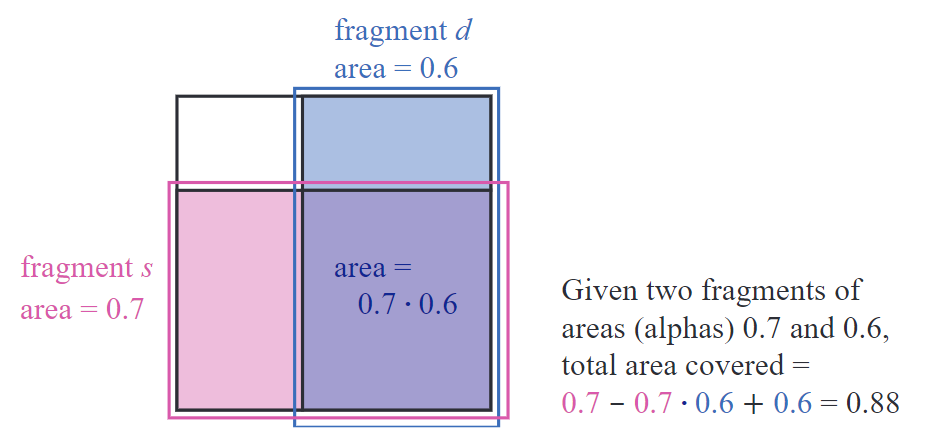#### 无序透明(Order-Independent Transparency)

under操作可以先将所有半透明物体渲染到一个单独的颜色buffer上，再用over操作与后面的不透明场景进行混合。它也可以用来执行无序透明算法(Order-independent Transparency, OIT)，即深度剥离(depth peeling)，这也就意味着无需进行排序。它需要使用两个z-buffer和多通道。它的步骤如下：

1. 使用一个渲染通道将所有物体表面（包括半透明物体）的深度值记录到第一个z-buffer中。
2. 在第二个通道中渲染所有的半透明物体。
3. 如果2中一个物体的深度值和第一个z-buffer中的一样，我们知道它是距离观察点最近的半透明物体，将它的RGBA存储到单独的一个颜色buffer中。
4. 寻找下一个半透明物体，它的深度值大于且最接近于上一步中的深度值，则将它的深度值记录下来，并将它的RGBA与上一步中的颜色buffer做under操作。
5. 重复第4步n次，直到找不到更远的半透明物体，或达到设定的通道上限。
6. 将上述步骤得到的半透明物体颜色buffer和不透明场景进行over混合。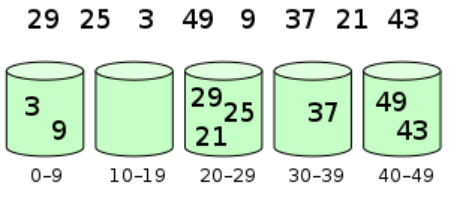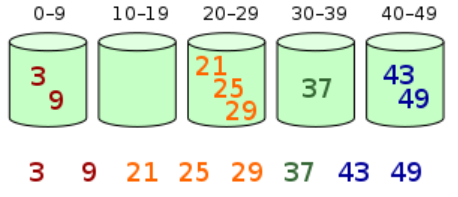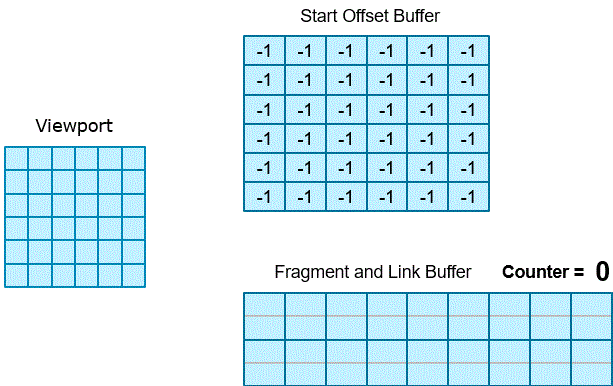A-buffer的优点是每个像素对应多少fragment就记录多少值，但这同样是个缺点，因为在渲染开始前并不知道需要存储多少内容。如果场景里面有大量烟雾、头发等物体，就会产生大量的fragment。

GPU通常会为buffer、数组等预先分配内存，链表也不例外，因此开发者需要提前规划好需要使用多少内存，否则就会出现内存不足的问题。多层alpha混合(multi-layer alpha blending, MLAB)就是为了解决这个问题的，它使用了Intel推出的一个GPU功能，叫做像素同步(pixel synchronization)。它的原理简单来说就是在内存不足时，先将深度值相差在一定范围内的fragment进行颜色混合，再存储到A-buffer中，这样可以减少A-buffer的存储量。这个“一定范围”可以根据内存进行调节。由于提前混合多个fragment时顺序未知，因此可能会出现一点小问题。但由于它们离得比较近，因此问题不大。当然，如果能进行一个粗略的排序就能获得更好的效果，比如ROV(rasterizer order views，详见第三章)。在移动端，也有类似MLAB的功能，叫做图块本地存储(tile local storage)。这种类型的算法对性能消耗比较大。

MLAB是基于k-buffer而来的。k-buffer的主要思路就是尽可能对前面的图层进行排序和存储，对后面的图层进行混合和丢弃。基于此，衍生出了Weighted Sum和Weighted Average算法。Weighted Sum算法公式如下：

$\mathbf{c}_o=\sum_{i=1}^{n}(\alpha_i\mathbf{c}_i)+\mathbf{c}_d(1-\sum_{i=1}^{n}\alpha_i)$

Weighted Average可以避免上述问题：

$\mathbf{c}_{\text{sum}}=\sum_{i=1}^{n}(\alpha_i\mathbf{c}_i),\;\;\;\alpha_{\text{sum}}=\sum_{i=1}^{n}\alpha_i$

$\mathbf{c}_{\text{wavg}}=\frac{\mathbf{c}_{\text{sum}}}{\alpha_{\text{sum}}},\;\;\;\alpha_{\text{avg}}=\frac{\alpha_{\text{sum}}}{n}$

$u=(1-\alpha_{\text{avg}})^n$

$\mathbf{c}_o=(1-u)\mathbf{c}_{\text{wavg}}+u\mathbf{c}_d$

#### 预乘Alpha和合成(Premultiplied Alphas and Compositing)

over操作还可以用来混合图片或合成物，也即合成(Compositing)，alpha值会和每个像素的RGB值一起被存储。由alpha通道形成的图片有时被称为遮罩(matte)，它显示了物体的轮廓信息。

$\mathbf{c}_o=\mathbf{c}_s'+(1-\alpha_s)\mathbf{c}_d$

$\mathbf{c}_s'=\alpha_s\mathbf{c}_s$